# Connecting a Servo Motor to An Arduino

Building robots with Arduino starts with knowing how to move objects through motors. There are a lot of types of motors used in microcontroller-based systems: DC motors, stepper motors, and servo motors.  In this Arduino servo motor article, I will discuss what is a servo motor and how to use it with an Arduino.

### Introduction to Servo Motors

A servo motor is a special device containing a DC motor, feedback electronics, and a set of gears. It follows the concept of servomechanism.

The operation of the servo motor can be described by the diagram below:• A servo motor accepts pulses and outputs rotation.
• When the DC motor is at a stop, it means the motor shaft and potentiometer knob are in their equilibrium position. The inputs to the error amplifier are equal and the motor is undriven.
• When a pulse is applied, the two inputs on the error amplifier are no longer equal and thus the amplifier drives the motor. As the motor turns, the gear assembly turns and thus also turns the potentiometer.
• The potentiometer (via voltage divider) generates a voltage equivalent to that of the input pulse that stops the DC motor, indicating equilibrium.

The gear assembly slows down the rotation of the motor to a speed that the potentiometer can catch up. Also, the gears boost the torque output of the servo motor.

#### Pulse Width and Position

The position of the servo motor arm corresponds with the width of the pulse:

The exact pulse width could vary per servo motor. If you want to get the exact pulse width for a specific angle, you must test your servo motor.

There is a mechanical stop at the gear assembly to limit the rotation of servo motors to 180 degrees. There are two reasons why servo motors don't rotate for a full circle:

1. Originally designed for converting rotational motion to linear motion, servo motors do not need full 360-degree rotation (see crank mechanism).
2. The limited possible values for PWM signals need to be mapped to a smaller range (of angles).

There is a way to make a continuous rotation servo which is shown in this tutorial. A continuous rotation servo is stronger than a an ordinary dc motor.

### Servo Motor Internals

Here is my MG996R servo motor: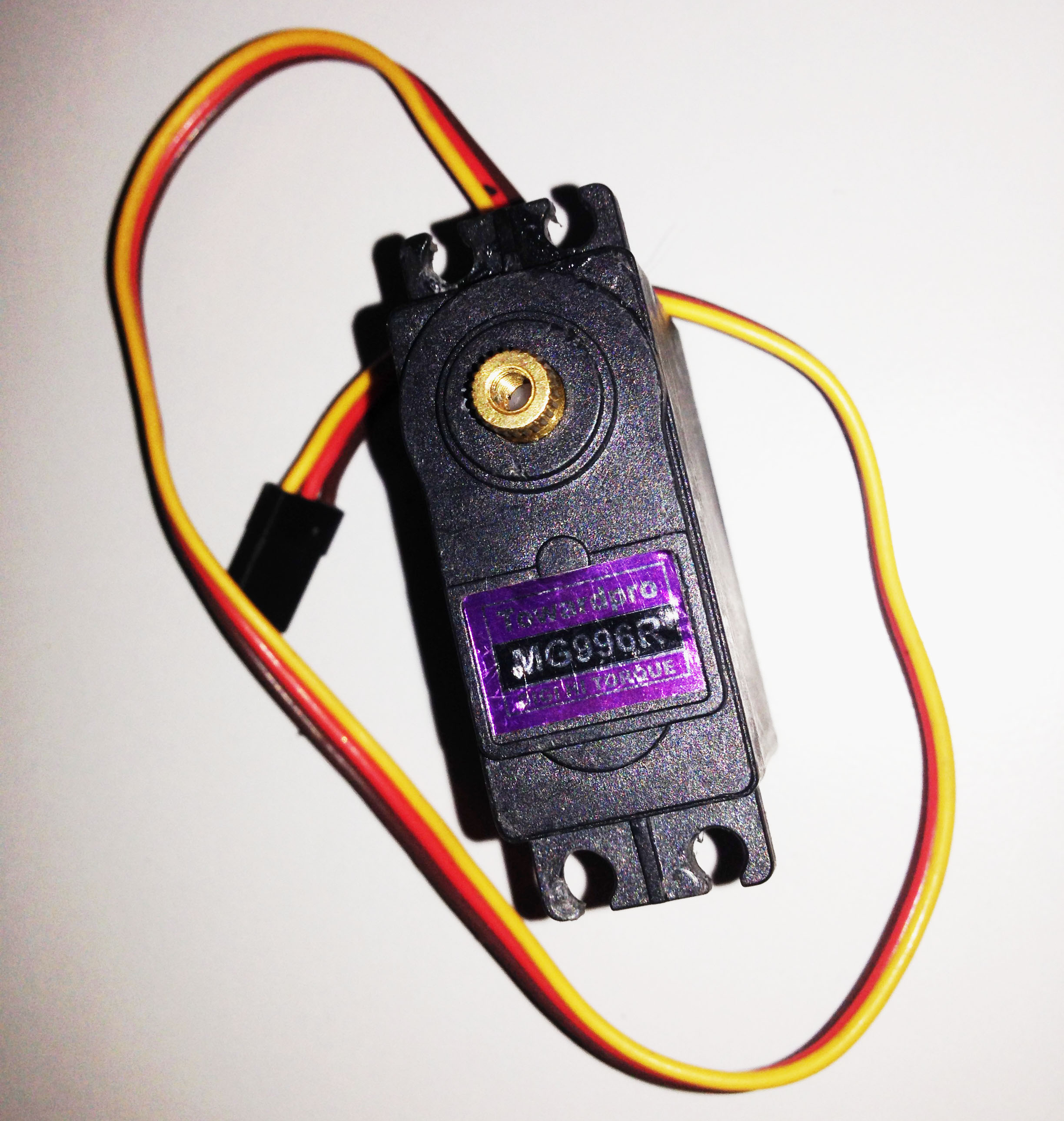As you can see, there are three wires: red for +5V, black for ground, and yellow for pulse. Other servo motor brands have different colors for their wires but almost all uses red for +5V and black for ground.

This servo motor is capable of producing high torque (rotational force), and thus has metal gears inside: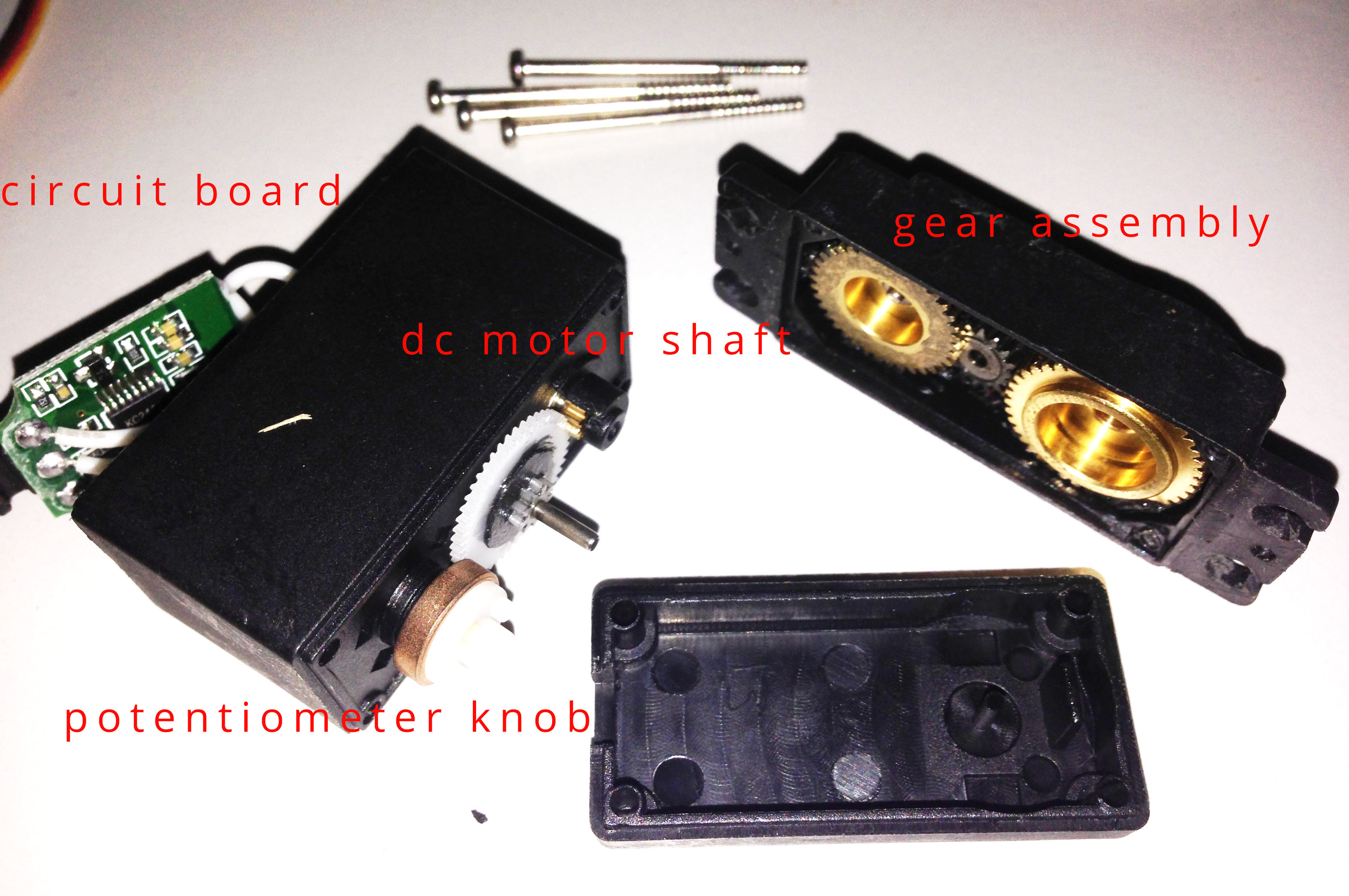Servo motors that don't produce high torque typically contain plastic gears.

A better look at the circuit board, DC motor and potentiometer: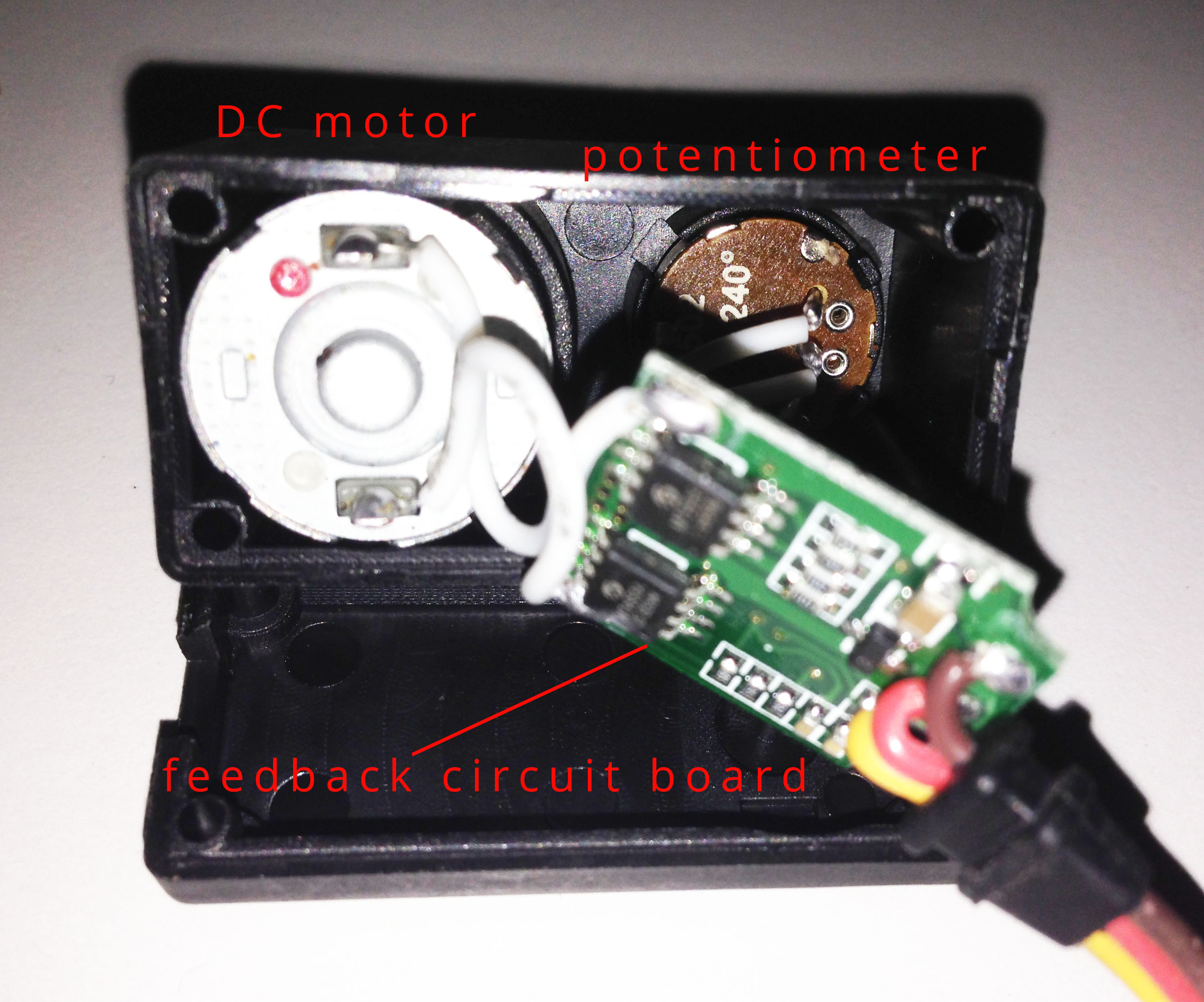### Arduino to Servo Motor Wiring Diagram

To begin, wire this circuit: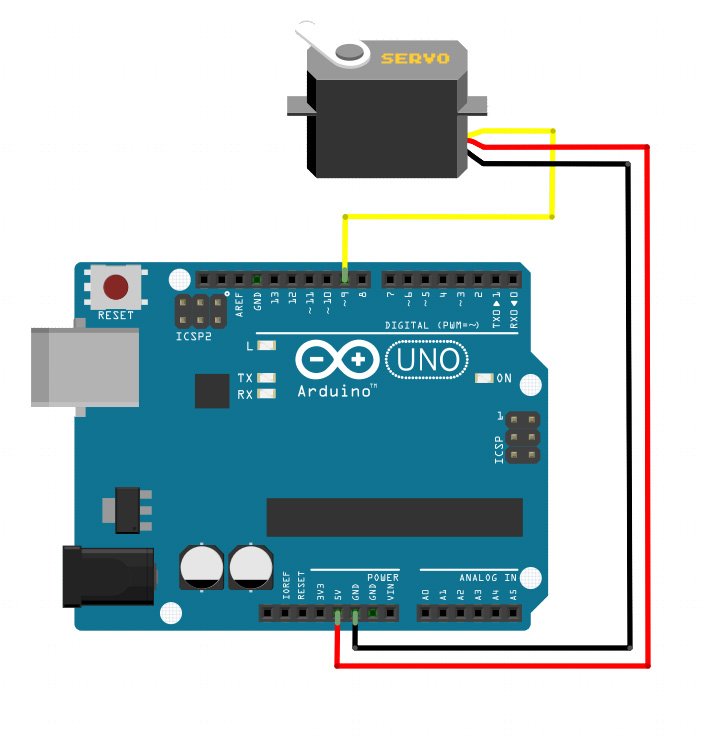Most servo motors run on 5V so you can attach the red lead to the Arduino’s +5V pin. However, larger servos might draw more current which can reset the Arduino.

My MG996R draws 10 mA at idle, 170 mA when operating but without any load connected, and stalls at 1400 mA (!).

Remember that the Arduino +5v pin is limited to 500 mA! Thus,  the number (and sometimes, type) of servos you can use is limited.

The yellow pin is where you would send PWM signals to the Arduino. This means this lead must be connected to any of the pins with the ~ in them.

Side note: But it doesn’t mean that the control pin is limited to only a PWM pin. By the way, there are other ways to generate PWM besides the hardware PWM on the Arduino.

### Programming an Arduino for Servomotors

The Arduino Servo library has two easy-to-use examples. This library comes with the IDE so no additional downloads are necessary. We’ll first be using the Sweep example. On the Arduino IDE, go to File > Examples > Servo > Sweep. This sketch will open up:

```#include <Servo.h>

Servo myservo; // create servo object to control a servo
// twelve servo objects can be created on most boards

int pos = 0; // variable to store the servo position

void setup()
{
myservo.attach(9); // attaches the servo on pin 9 to the servo object
}

void loop()
{
for(pos = 0; pos <= 180; pos += 1) // goes from 0 degrees to 180 degrees
{ // in steps of 1 degree
myservo.write(pos); // tell servo to go to position in variable 'pos'
delay(15); // waits 15ms for the servo to reach the position
}
for(pos = 180; pos>=0; pos-=1) // goes from 180 degrees to 0 degrees
{
myservo.write(pos); // tell servo to go to position in variable 'pos'
delay(15); // waits 15ms for the servo to reach the position
}
}```

#### Dissecting the Sketch

Of course, the code starts with

`#include <Servo.h>`

Which simply tells the compiler to include all the Arduino Servo library functions.

Next, you must create a Servo object like this:

`Servo myservo;`

According to the creators of the Servo library, you can create up to 12 servo objects for the UNO and most other boards and up to 48 for the Arduino Mega.

You then need to attach the Servo object to a pin:

`myservo.attach(9);`

To move the servo arm, you use the servo.write() function like so:

```for(pos = 0; pos <= 180; pos += 1) // goes from 0 degrees to 180 degrees
{ // in steps of 1 degree
myservo.write(pos); // tell servo to go to position in variable 'pos'
delay(15); // waits 15ms for the servo to reach the position
}

for(pos = 180; pos>=0; pos-=1) // goes from 180 degrees to 0 degrees
{
myservo.write(pos); // tell servo to go to position in variable 'pos'
delay(15); // waits 15ms for the servo to reach the position
}```

The servo.write() function receives the angle in degrees as the argument. In this code snippet, pos is incremented from 0 to 180 inside a for loop and then decremented from 180 to 0 in another for loop.

#### Using Microseconds instead of Angles

If you want to write microseconds for controlling instead of degrees, you can use the writeMicroseconds() function. This is a common example that positions the servo motor arm at the center:

```#include <Servo.h>

Servo myservo;

void setup()
{
myservo.attach(9);
myservo.writeMicroseconds(1500);  // set servo to mid-point
}

void loop() {}
```

Here, a 1500 microsecond pulse is generated by the Arduino which is equivalent to 1.5 milliseconds, the pulse width for centering the arm of the servo motor.

### Use a Potentiometer to Control a Servo Motor

The other example, the Knob sketch, shows how to control a servo motor with a potentiometer. The voltage from the potentiometer circuit is read through analogWrite and converted to rotation. I used this wiring diagram for this next example: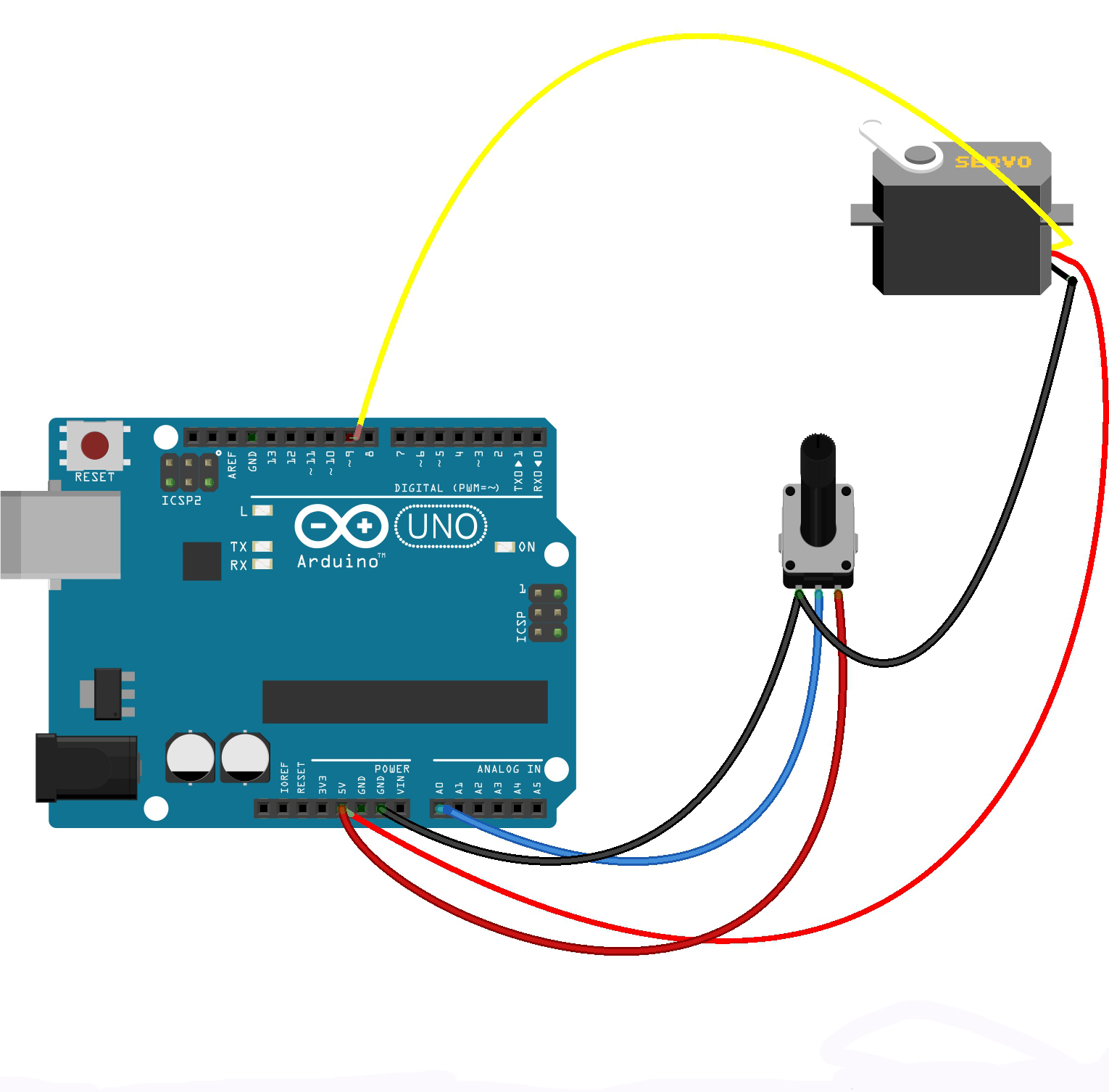#### Knob Sketch:

```#include <Servo.h>

Servo myservo;  // create servo object to control a servo

int potpin = 0;  // analog pin used to connect the potentiometer
int val;    // variable to read the value from the analog pin

void setup() {
myservo.attach(9);  // attaches the servo on pin 9 to the servo object
}

void loop() {
val = analogRead(potpin);            // reads the value of the potentiometer (value between 0 and 1023)
val = map(val, 0, 1023, 0, 180);     // scale it to use it with the servo (value between 0 and 180)
myservo.write(val);                  // sets the servo position according to the scaled value
delay(15);                           // waits for the servo to get there
}```

Similar to the Sweep sketch, the servo motor signal pin is attached to pin 9 of the Arduino UNO. The wiper (middle) arm of the potentiometer is connected to analog pin 0 while the other pins are connected to +5 and GND. With this connection, the potentiometer acts as a voltage divider circuit.

When the wiper arm of the potentiometer is turned, the voltage on the A0 pin changes. Moreover, the minimum value is zero while the maximum value read by the analogRead() function is 1023 according to ADC theory. This range of values is converted to degrees of rotation using the map() function.

### Multiple Servo Connections

It's more fun to use more servo motors! However, there are challenges. You cannot use the Arduino's +5V pin to power three or more servo motors because of the current limits of the board. The solution is to have a separate power source for the servo motors. Here is an example diagram:The six servo motors are powered by a 4.8 V battery (rechargeable) pack.

#### Sketch for Multiple Servo Motors

An example code for testing out multiple servos:

```#include <Servo.h>

Servo servo1, servo2, servo3, servo4, servo5, servo6;  // create servo objects to control a servo

void setup() {
Serial.begin(9600);

servo1.attach(3);
servo2.attach(5);
servo3.attach(6);
servo4.attach(9);
servo5.attach(10);
servo6.attach(11);
}

void loop() {

if (Serial.available())  {
char c = Serial.read();  //gets one byte from serial buffer
if (c == ',') {
Serial.println(readString); //prints string to serial port out

// auto select appropriate value, copied from someone elses code.
if(n >= 500)
{
Serial.print("writing Microseconds: ");
Serial.println(n);
}
else
{
Serial.print("writing Angle: ");
Serial.println(n);
}
readString=""; //clears variable for new input
}
}
else {